The perfect place for easy learning...

×

# What is Decision Making Statement?

In the C programming language, the program execution flow is line by line from top to bottom. That means the c program is executed line by line from the main method. But this type of execution flow may not be suitable for all the program solutions. Sometimes, we make some decisions or we may skip the execution of one or more lines of code. Consider a situation, where we write a program to check whether a student has passed or failed in a particular subject. Here, we need to check whether the marks are greater than the pass marks or not. If marks are greater, then we decide that the student has passed otherwise failed. To solve such kind of problems in c we use the statements called decision making statements.

Decision-making statements are the statements that are used to verify a given condition and decide whether a block of statements gets executed or not based on the condition result.

In the c programming language, there are two decision-making statements they are as follows.

1. if statement
2. switch statement

# if statement in c

In c, if statement is used to make decisions based on a condition. The if statement verifies the given condition and decides whether a block of statements are executed or not based on the condition result. In c, if statement is classified into four types as follows...

1. Simple if statement
2. if-else statement
3. Nested if statement

# Simple if statement

Simple if statement is used to verify the given condition and executes the block of statements based on the condition result. The simple if statement evaluates specified condition. If it is TRUE, it executes the next statement or block of statements. If the condition is FALSE, it skips the execution of the next statement or block of statements. The general syntax and execution flow of the simple if statement is as follows.Simple if statement is used when we have only one option that is executed or skipped based on a condition.

# Example Program | Test whether given number is divisible by 5.

``````#include<stdio.h>
#include<conio.h>

void main(){
int n ;
clrscr() ;
printf("Enter any integer number: ") ;
scanf("%d", &n) ;
if ( n%5 == 0 )
printf("Given number is divisible by 5\n") ;
printf("statement does not belong to if!!!") ;
}```
```

Output 1: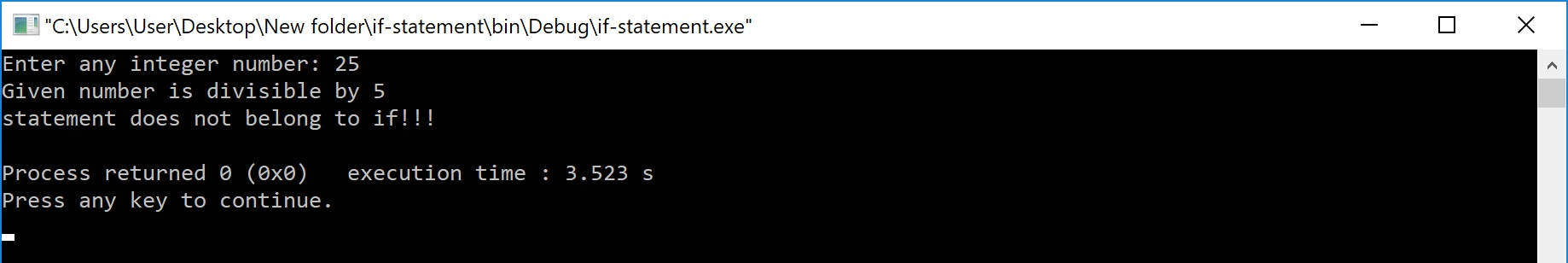Output 2: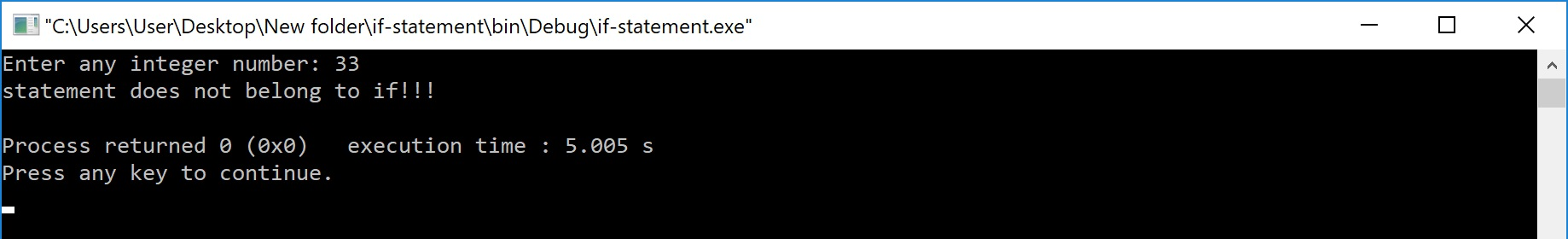# if-else statement

The if-else statement is used to verify the given condition and executes only one out of the two blocks of statements based on the condition result. The if-else statement evaluates the specified condition. If it is TRUE, it executes a block of statements (True block). If the condition is FALSE, it executes another block of statements (False block). The general syntax and execution flow of the if-else statement is as follows.The if-else statement is used when we have two options and only one option has to be executed based on a condition result (TRUE or FALSE).

# Example Program | Test whether given number is even or odd.

``````#include<stdio.h>
#include<conio.h>

void main(){
int n ;
clrscr() ;
printf("Enter any integer number: ") ;
scanf("%d", &n) ;
if ( n%2 == 0 )
printf("Given number is EVEN\n") ;
else
printf("Given number is ODD\n") ;
}```
```

Output 1: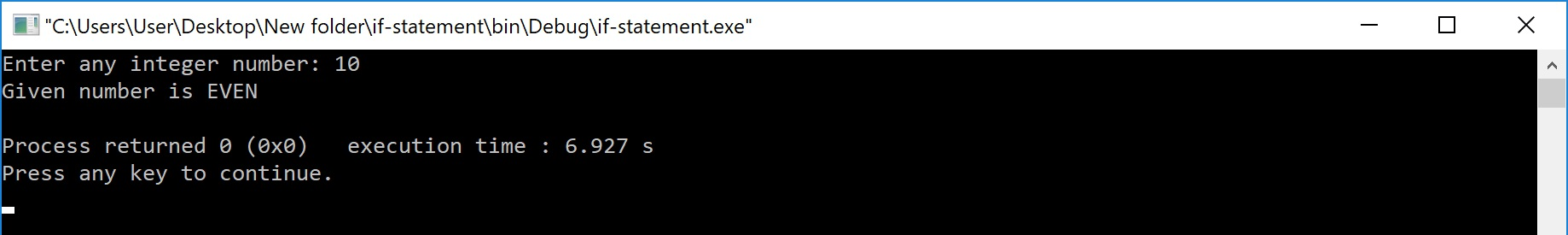Output 2: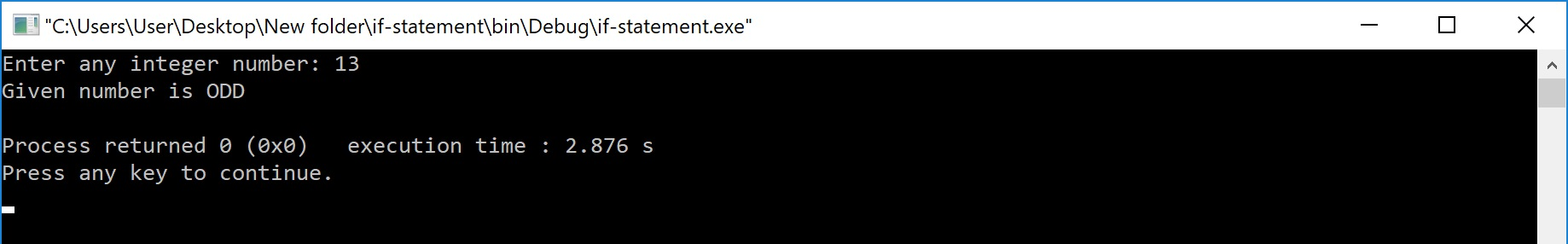# Nested if statement

Writing a if statement inside another if statement is called nested if statement. The general syntax of the nested if statement is as follows...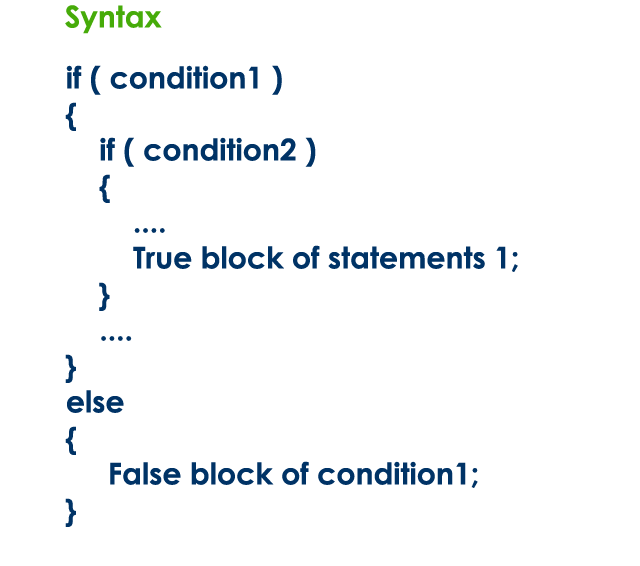The nested if statement can be defined using any combination of simple if & if-else statements.

# Example Program | Test whether given number is even or odd if it is below 100.

``````#include<stdio.h>
#include<conio.h>

void main(){
int n ;
clrscr() ;
printf("Enter any integer number: ") ;
scanf("%d", &n) ;
if ( n < 100 )
{
printf("Given number is below 100\n") ;
if( n%2 == 0)
printf("And it is EVEN") ;
else
printf("And it is ODD") ;
}
else
printf("Given number is not below 100") ;
}```
```

Output 1: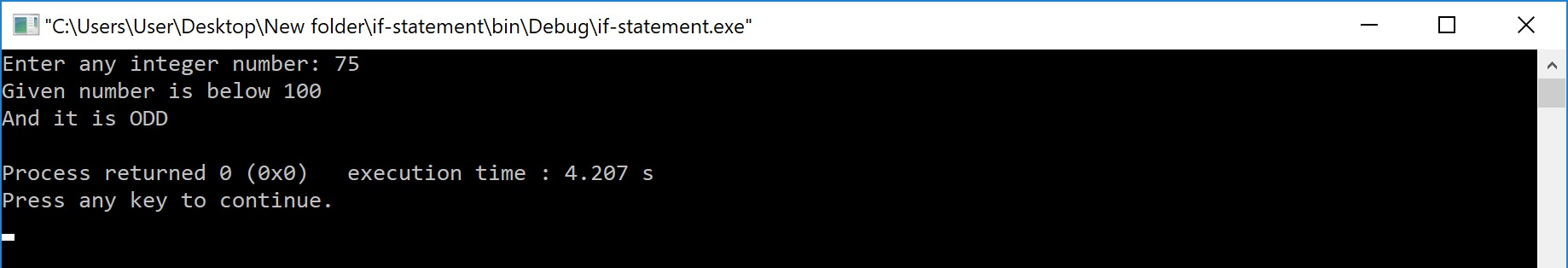Output 2:Writing a if statement inside else of an if statement is called if-else-if statement. The general syntax of the if-else-if statement is as follows...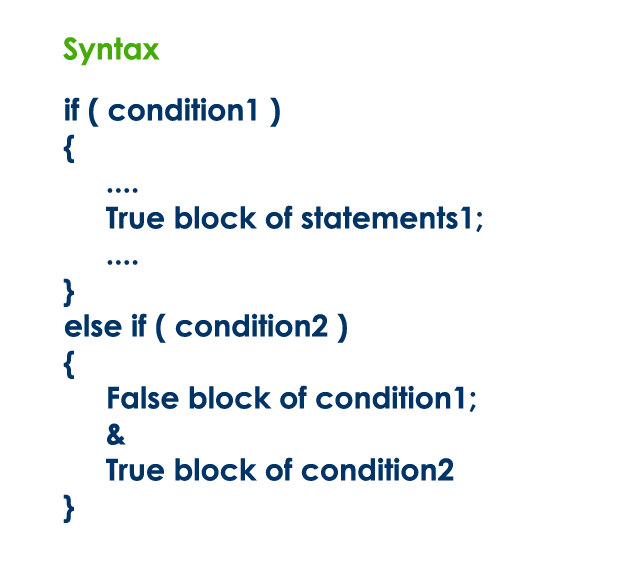The if-else-if statement can be defined using any combination of simple if & if-else statements.

# Example Program | Find the largest of three numbers.

``````#include<stdio.h>
#include<conio.h>

void main(){
int a, b, c ;
clrscr() ;

printf("Enter any three integer numbers: ") ;
scanf("%d%d%d", &a, &b, &c) ;

if( a>=b && a>=c)
printf("%d is the largest number", a) ;

else if (b>=a && b>=c)
printf("%d is the largest number", b) ;

else
printf("%d is the largest number", c) ;
}```
```

Output: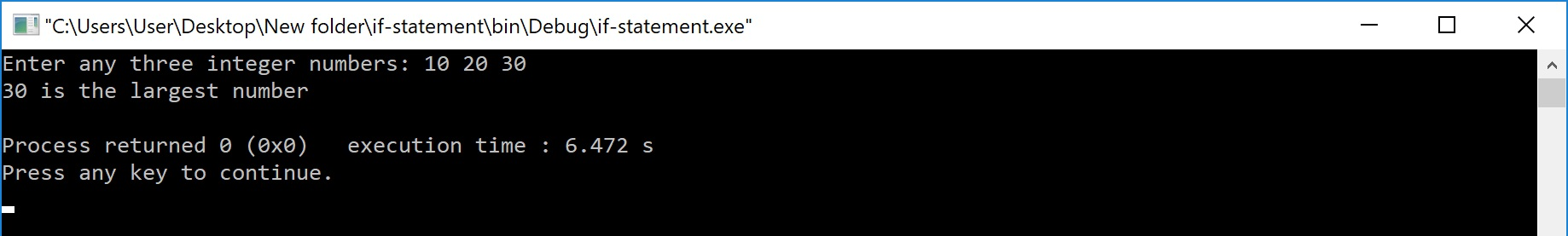MOST IMPORTANT POINTS TO BE REMEMBERED

When we use a conditional control statement like if statement, the condition might be an expression evaluated to a numerical value, a variable or a direct numerical value. If the expression value or direct value is zero the condition becomes FALSE otherwise becomes TRUE.

To understand more consider the following statements.

• if(10) - is TRUE
• if(x) - is FALSE if x value is zero otherwise TRUE
• if(a+b) - is FALSE if a+b value is zero otherwise TRUE
• if(a = 99) - is TRUE because a value is non-zero
• if(10, 5, 0) - is FALSE because it considers last value
• if(0) - is FALSE
• if(a=10, b=15, c=0) - is FALSE because last value is zero